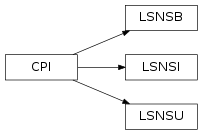# 5.9. `pyopus.problems.karmitsa` — Large scale nonsmooth test functions (Karmitsa set)¶Large scale nonsmooth test functions (Karmitsa set) (PyOPUS subsystem name: KARMITSA)

This module is independent of PyOPUS, meaning that it can be taken as is and used as a module in some other package. It depends only on the `cpi` and the `_karmitsa` modules.

 [karmitsa] Karmitsa N.: Test Problems for Large-Scale Nonsmooth Minimization, Technical report B.4/2007, University of Jyvaskyla, Jyvaskyla, 2007.
class `pyopus.problems.karmitsa.``LSNSU`(name=None, number=None, n=2)

Unconstrained problems from the Karmitsa test suite. Problems 10 and 11 were added by Á. Bűrmen.

• name - problem name
• number - problem number (0-11)
• n - problem dimension

Attributes:

• `name` - problem name
• `n` - number of variables
• `initial` - initial values of variables
• `fmin` - best known minimum

This module is independent of PyOPUS, meaning that it can be taken as is and used as a module in some other package. It depends only on the cpi and the _karmitsa modules.

`cpi`()

Returns the common problem interface.

Subgradient is not supported.

xmin is also not available.

See the `CPI` class for more information.

`f`(x)

Returns the value of the function at x.

`names` = ['GeneralizedMAXQ', 'GeneralizedMXHILB', 'ChainedLQ', 'ChainedCB3I', 'ChainedCB3II', 'ActiveFaces', 'GeneralizedBrown2', 'ChainedMifflin2', 'ChainedCrescentI', 'ChainedCrescentII', 'GeneralizedL1HILB', 'GeneralizedWatson']

List of all function names

class `pyopus.problems.karmitsa.``LSNSB`(name=None, number=None, n=2, feasible=1)

Bound constrained problems from the Karmitsa test suite.

• name - problem name
• number - problem number (0-9)
• n - problem dimension
• feasible - 0=initial point unchanged, 1=feasible, 2=strictly feasible, default=1

Attributes:

• `name` - problem name
• `n` - number of variables
• `xl` - lower bounds on variables
• `xh` - upper bounds on variables
• `initial` - initial values of variables
• `fmin` - best known minimum

This module is independent of PyOPUS, meaning that it can be taken as is and used as a module in some other package. It depends only on the cpi and the _karmitsa modules.

`cpi`()

Returns the common problem interface.

Subgradient is not supported.

xmin is also not available.

See the `CPI` class for more information.

`f`(x)

Returns the value of the function at x.

`names` = ['GeneralizedMAXQ', 'GeneralizedMXHILB', 'ChainedLQ', 'ChainedCB3I', 'ChainedCB3II', 'ActiveFaces', 'GeneralizedBrown2', 'ChainedMifflin2', 'ChainedCrescentI', 'ChainedCrescentII']

List of all function names

class `pyopus.problems.karmitsa.``LSNSI`(name=None, number=None, cname=None, cnumber=None, n=10)

Inequality constrained problems from the Karmitsa test suite.

• name - function name
• number - function number (0-9)
• cname - constraint name
• cnumber - constraint number (0-7)
• n - problem dimension

Attributes:

• `name` - problem name
• `fname` - function name
• `cname` - constraint name
• `n` - number of variables
• `m` - number of constraints
• `cl` - lower bounds on constraint functions
• `ch` - upper bounds on constraint functions
• `initial` - initial values of variables

This module is independent of PyOPUS, meaning that it can be taken as is and used as a module in some other package. It depends only on the cpi and the _karmitsa modules.

`c`(x)

Returns the value of the constraints at x.

`cnames` = ['TridiagonalI', 'TridiagonalII', 'MAD1I', 'MAD1II', 'ModifiedMAD1I', 'ModifiedMAD1II', 'P20I', 'P20II']

List of all constraint names

`cpi`()

Returns the common problem interface.

Subgradient is not supported.

xmin is also not available.

See the `CPI` class for more information.

`f`(x)

Returns the value of the function at x.

`names` = ['GeneralizedMAXQ', 'GeneralizedMXHILB', 'ChainedLQ', 'ChainedCB3I', 'ChainedCB3II', 'ActiveFaces', 'GeneralizedBrown2', 'ChainedMifflin2', 'ChainedCrescentI', 'ChainedCrescentII']

List of all function names

Example file karmitsa.py in folder demo/problems/

```# Karmitsa test suite

from pyopus.problems.karmitsa import *

if __name__=='__main__':
print("Unconstrained, n=50")
for ii in range(10):
prob=LSNSU(number=ii, n=50)
print("%d: %25s: f0=%e" % (ii, prob.name, prob.f(prob.initial)))
print()

print("Bound constrained, n=50")
for ii in range(10):
prob=LSNSB(number=ii, n=50)
print("%d: %25s: f0=%e" % (ii, prob.name, prob.f(prob.initial)))
print()

print("Inequality constrained, n=10")
for ii in range(10):
for jj in range(8):
prob=LSNSI(number=ii, cnumber=jj, n=10)
c=prob.c(prob.initial)
feas=((c>prob.cl) & (c<prob.ch)).all()
print("%d, %d: %30s: f0=%12.4e feas=%d" % (ii,jj, prob.name, prob.f(prob.initial), feas))
print()

```